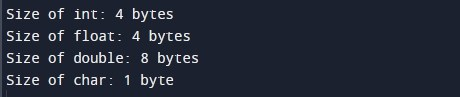C Program to Print Size of int, float, double and char

In this tutorial you will learn about the C Program to Print Size of int, float, double and char and its application with practical example.

In this tutorial, we will learn to create a program that finds the size of various data types such as int, float, double and char in C programming.

Prerequisites

Before starting with this tutorial we assume that you are best aware of the following C programming topics:

• C Data Types
• C Variables
• C Input Output

Program to Print Size of int, float, double and char

In this program we will find the size of various data types such as int, float, double and char using sizeof operator. In C Programming, the sizeof operator returns the amount of the memory allocated to a variable of specific datatype such as int, float, double and char. In this program we will first declare and initialize a set variables each one of different data type. Later in the program we will display size of various data types such as int, float, double and char.

Output:-In the above program, we have first declared and initialized a set variables required in the program.

• intVar = it is a variable of integer data type
• floatVar = it is a variable of float data type
• doubleVar = it is a variable of double data type
• charVar = it is a variable of char data type

Later in the program we will find the size of various data types such as int, float, double and char using sizeof operator and will print using printf statement.

In this tutorial we have learn about the C Program to Print Size of int, float, double and char and its application with practical example. I hope you will like this tutorial.